Courses

# Surveying (Part - 12) Civil Engineering (CE) Notes | EduRev

## Civil Engineering (CE) : Surveying (Part - 12) Civil Engineering (CE) Notes | EduRev

The document Surveying (Part - 12) Civil Engineering (CE) Notes | EduRev is a part of the Civil Engineering (CE) Course Civil Engineering SSC JE (Technical).
All you need of Civil Engineering (CE) at this link: Civil Engineering (CE)

Surveying (Part 12)

Two cases may arise

(I) An observation is made on the bright portion
(II) An observation is made on the bright line

(I) When the observation is made on the bright portion
b = Phase correction angel COP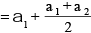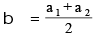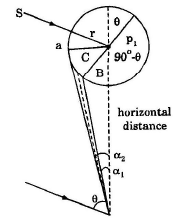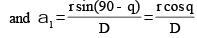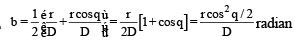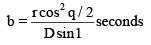where r = radius of the signal b = angle between sun and the line OP

II. When the observation is made on the Bright line Ð SCO = 180 (q -b)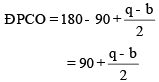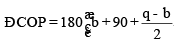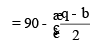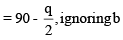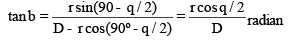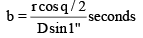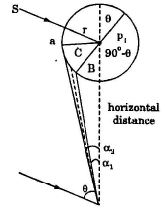The phase correction is applied algebrically to the observed angle according to the relative positions of the sun and the signal, i.e., it is the +ve and zero.
Measurement of Base line The accuracy of any order of triangulation depends upon the accuracy of the measurement of its base line.
Hence in triangulation a base line is of prime importance.

Selection of the site for a Base line
1. The ground of the sight should be fairly level or uniformal.

Number of zeros: To eliminate the error due to inaccurate graduatios of the horizontal circle, the measures of the horizontal angles are taken in two or three different zones, i.e.,

Type of  Triangulations stations

(i) Main station
(ii) Subsidiary stations
(iii) Satellite stations or Eccentric or false stations
(iv) Pivot stations

Signals and Towers
(a) Luminous signal, and
(b) Opaque signals or non-luminous signal

• Base line is measurred by standard tape and after that following connections are applied to the measured base length.
(1) Correction for standard length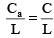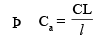where L = measured length of the line
l = designed length of the tape
C= correction fro absolute length
C = correction to be applied to the tape.

The sign of the correction Ca will be the same as that of C.

(2) Correction of Alignment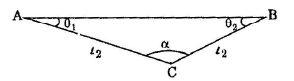Cn = (l1+l2) - (l1 cos q1 + l2 cos q2) = l1 (1– cos q1)+l2 (1–cosq2).

It is always substractive If A and B are not intervisible, then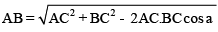(3) Correction for shope Cs = L – D

= L – (L2–b2)1/2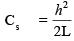It is always substractive

or Slope correction = L–L cos q = L (1– cosq)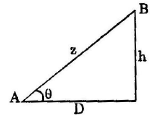4) Correction for Tension :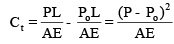where,P= standard pull P = Pull applied during measurement

If applied pull in more, tension correction is + ve, and if it is less, tension correction is ‘–’ ve

Offer running on EduRev: Apply code STAYHOME200 to get INR 200 off on our premium plan EduRev Infinity!

## Civil Engineering SSC JE (Technical)

109 docs|50 tests

,

,

,

,

,

,

,

,

,

,

,

,

,

,

,

,

,

,

,

,

,

;